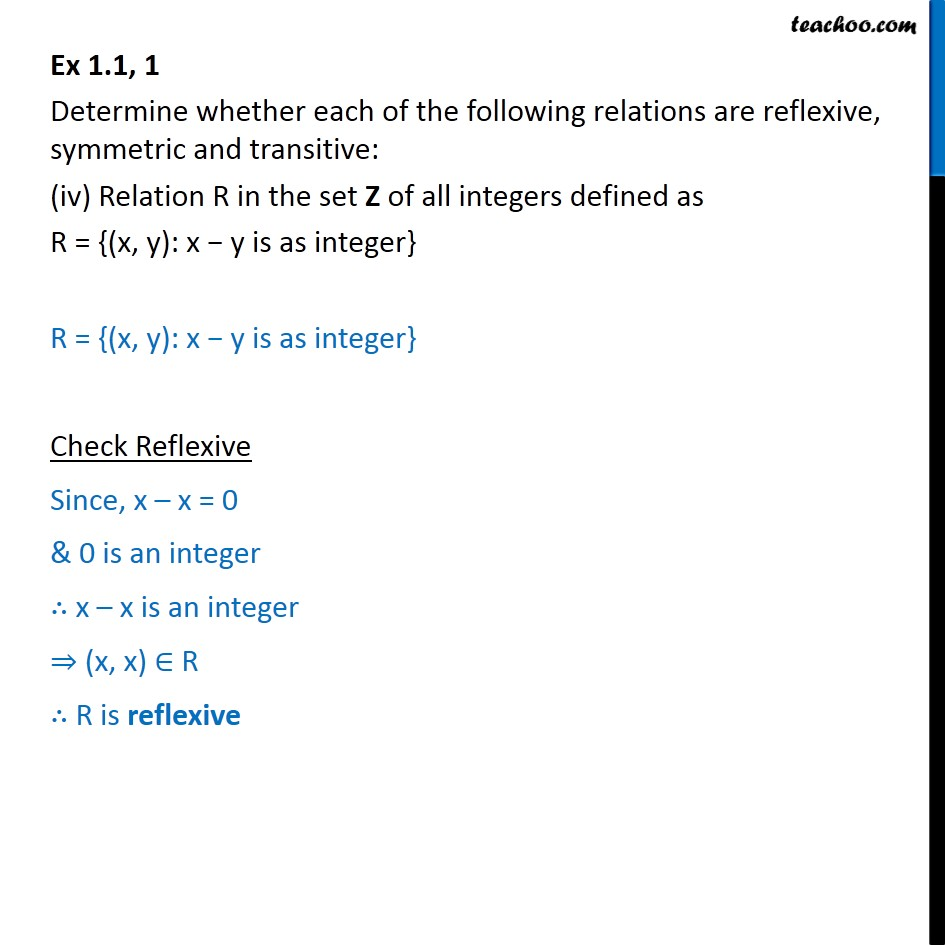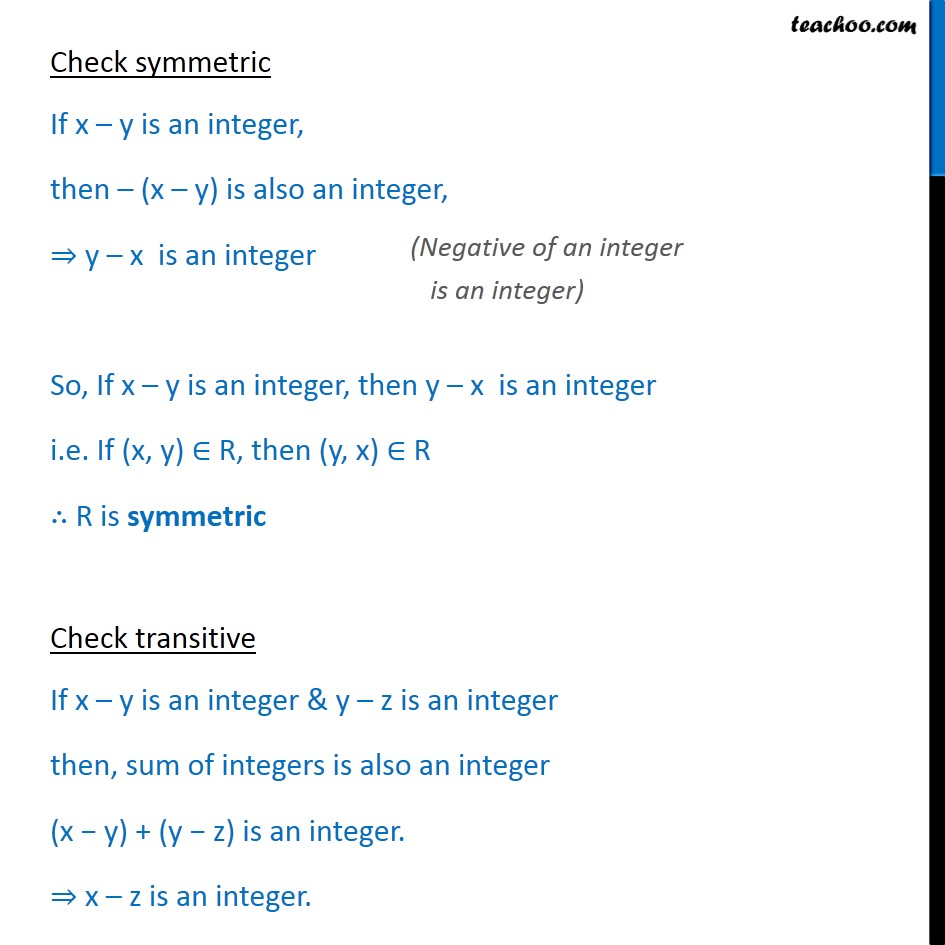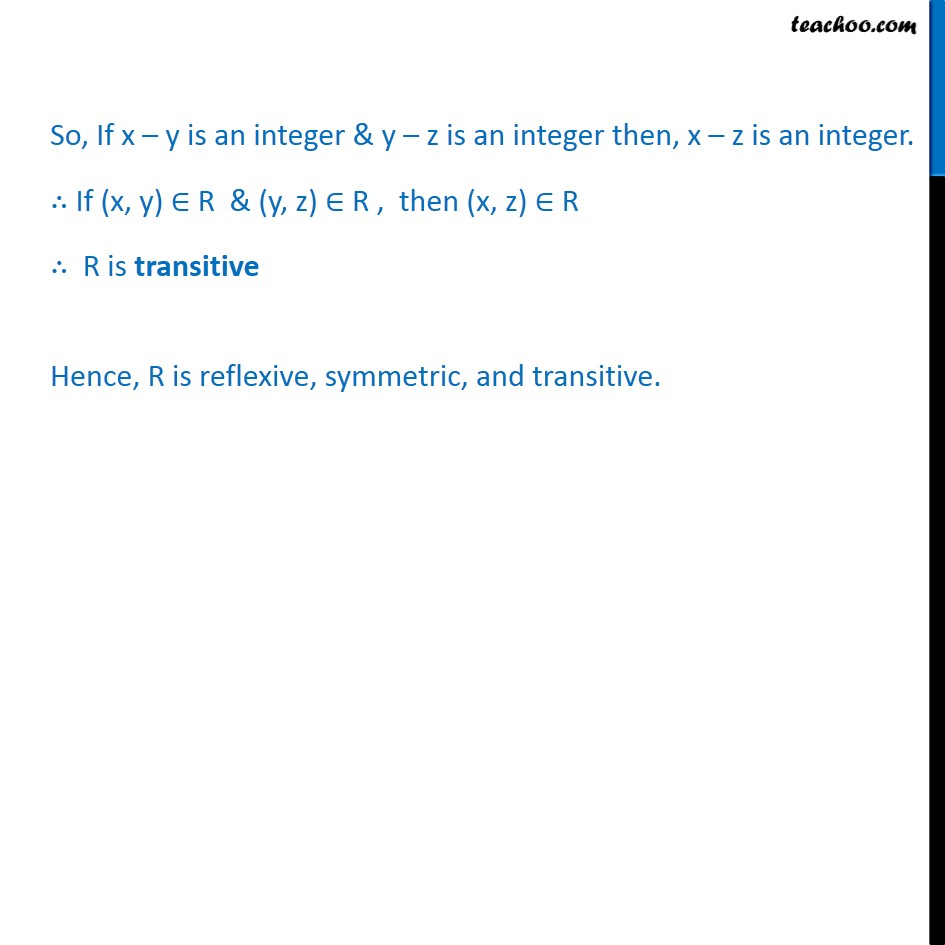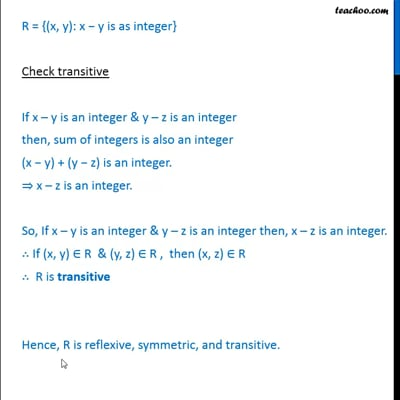Ex 1.1

Chapter 1 Class 12 Relation and Functions
Serial order wiseThis video is only available for Teachoo black users

Introducing your new favourite teacher - Teachoo Black, at only ₹83 per month

### Transcript

Ex 1.1, 1 Determine whether each of the following relations are reflexive, symmetric and transitive: (iv) Relation R in the set Z of all integers defined as R = {(x, y): x y is as integer} R = {(x, y): x y is as integer} Check Reflexive Since, x x = 0 & 0 is an integer x x is an integer (x, x) R R is reflexive Check symmetric If x y is an integer, then (x y) is also an integer, y x is an integer So, If x y is an integer, then y x is an integer i.e. If (x, y) R, then (y, x) R R is symmetric Check transitive If x y is an integer & y z is an integer then, sum of integers is also an integer (x y) + (y z) is an integer. x z is an integer. So, If x y is an integer & y z is an integer then, x z is an integer. If (x, y) R & (y, z) R , then (x, z) R R is transitive Hence, R is reflexive, symmetric, and transitive.